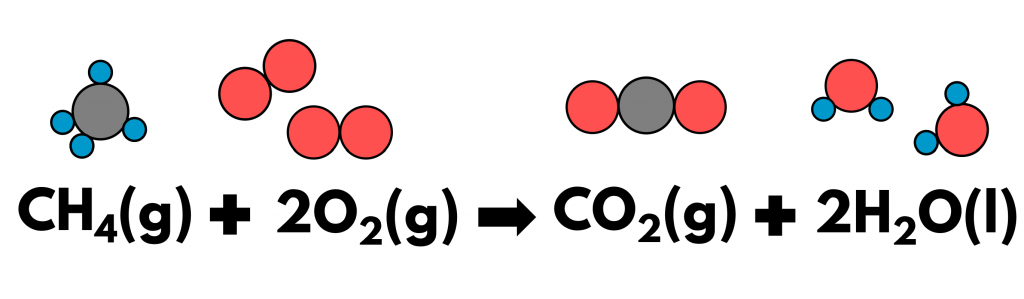## 1. Spoiler alert: chemical equations tell us everything about a reaction

Firstly, the chemical formulae in an equation tell us what we started with and are left with at the end.

• Reactants: hydrogen and oxygen
• Product: water

Secondly, the symbol in the brackets tells us which state our reactants and products are in:

Thirdly, the coefficients, which are the number before chemical formulae, tell us how much of each substance is reacted or produced, relative to each other.

• The coefficient of 2 before hydrogen means that for every molecule of oxygen that reacts, 2 molecules of hydrogen are needed
• The coefficient of 2 before water means that for every molecule of oxygen that has reacted, 2 molecules of water

## 2. The number of atoms does not change during a reaction

The law of conservation of mass implies that atoms are neither created nor destroyed during a chemical reaction.

In other words, a chemical reaction is simply an epic rearrangement of atoms. Amidst this drama, the number of atoms of each element remains the same.

## 3. Balance a chemical equation with coefficient

This is why we have to balance chemical equations, by writing coefficients such that the number of atoms before and after a reaction remains the same.Combustion of methane: the blue balls represent hydrogen atoms, the grey balls carbon atoms, the red balls oxygen atoms

The above equation showing the combustion of methane is balanced, because:

• While the carbon atom in methane is recombined with oxygen to form carbon dioxide, the number of carbon atom remains as 1.
• While the hydrogen atoms in methane is rearranged to form water, the number of hydrogen remains as 4.
• While the oxygen atoms in oxygen molecules are rearranged to form carbon dioxide and water, the number of oxygen atoms remains as 4.

## 3. Exam strategy to balance chemical equations

To practise, let’s consider the formation of ammonia gas from nitrogen gas and hydrogen gas.

• ### STEP 1: Identify the reactants and products

This piece of information is usually given by the question, so read carefully. If the reaction is a common one, then you will be required to recall from memory. Here, we have:

Nitrogen + Hydrogen ⟶ Ammonia

• ### STEP 2: Write the chemical formulae of the reactants and products

For simple molecules, you will have to recall from memory. For ionic compounds, you can infer the formulae based on the charges of the constituent ions.

N2 + H2 ⟶ NH3

• ### STEP 3: Balance by adding coefficients

Add coefficients before formulae to balance the equation.

N2 + 3H22NH3